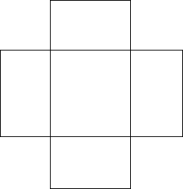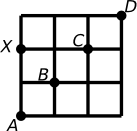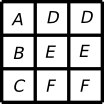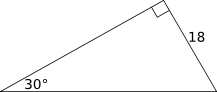#Solutions for Practice Test 4, The Official SAT Study Guide, Section 3

Math Lair Home > Test Preparation > Solutions for Practice Test 4, The Official SAT Study Guide, Section 3
SAT Practice Test Solutions:
2014–15 SAT Practice Test
2013–14 SAT Practice Test
The Official SAT Study Guide, second edition
• Practice Test 1: Sections 3, 7, 8.
• Practice Test 2: Sections 2, 5, 8.
• Practice Test 3: Sections 2, 5, 8.
• Practice Test 4: Sections 3, 6, 9.
• Practice Test 5: Sections 2, 4, 8.
• Practice Test 6: Sections 2, 4, 8.
• Practice Test 7: Sections 3, 7, 9.
• Practice Test 8: Sections 3, 7, 9.
• Practice Test 9: Sections 2, 5, 8.
• Practice Test 10: Sections 2, 5, 8.
SAT Math Tips

Here are solutions for section 3 of the fourth practice test in The Official SAT Study Guide, second edition, found on pages 581–586. The following solutions demonstrate faster, more informal methods that might work better for you on a fast-paced test such as the SAT. To learn more about these methods, see my e-book Succeeding in SAT Mathor the SAT math tips page.

1. Guess and check: Since 3b + 1 < 10, probably the largest value of b would make the expression false. So, try (E) 3. 3(3) + 1 = 10, which is not < 10. Select (E) 3.
2. Solution 1: Guess and check: Try each answer until you find one that works. Say that you started with (B) 2. 24 · 2 = 28 = 256, so the answer must be smaller. Try (A) 1. 24 · 1 = 16. So, that is the correct answer. Select (A) 1.

Solution 2: You may know (or could determine) that 24 = 16. Since we are given that 24x = 16, then 4 = 4x, or x = 1. Select (A) 1.

3. Solution 1: Try a special case: Say that r = 2. Then the question would become "How much greater than 0 is 7?" The answer, of course, is 7. Select (E) 7.

Solution 2: Convert the sentence into an equation:
 How much x (or another variable) greater than + r − 2 r − 2 is = r + 5 r + 5
The resulting equation is:

x + r − 2 = r + 5
x = 7
Select (E) 7.
• Draw a diagram: You may be able to visualize this in your head, but drawing a diagram helps to ensure that you get it correct. If you're right-handed, it may help to cover up the answers while you draw the diagram on the right side of the page. The resulting shape should consist of a big (4 × 3) rectangle (the bottom of the box), surrounded by four smaller rectangles (the sides). Your diagram should look something like the following:• Look at the answer choices: The only choice looking anything like the diagram is (E). Select that answer.
•Probably the best way to find the answer is by playing around a bit. Say that you start by moving up. Your next move must be up again; otherwise you'll run into B. This takes you to the point labelled X in the diagram on the right.
• Now, from point X, you have two options: Move up and then right, or move right and then up. After that, there are no other choices. So, there are a total of two ways if you start by moving up.
• By symmetry, there must be two ways of reaching the exit if you start by moving right. So, there are 2 + 2 = 4 ways in all. Select (B) Four.
4. Solution 1:
• Estimate the answer: If the question asked you to find 6/7 of n, the answer would be easy to find: It would be twice 42, or 84. However, you are asked to find 5/7 of n. This answer would have to be less than 84, but it would be closer to 6/7 of n = 84 than 3/7 of n = 42. So, the answer should be in the 60s or 70s.
• Look at the answer choices: The only answer anywhere near our estimate is (A) 70. Select that answer.
Solution 2:
• Convert the sentence into an equation:  If [ignore] 3/7 3/7 of × n n is = 42 42
This results in:
(3/7)n = 42
• We are asked to find 5/7 of n. We can obtain this number by multiplying both sides of the equation by 5/3:
(5/3)(3/7)n = (5/3)(42) (5/7)n = 70
Select (A) 70.
5. Solution 1:
• Read the question and understand what it is asking: The big rectangles in the box are made up of two little squares. You need to find what fraction of the box F is.
•Draw a diagram: The big rectangles can be divided into two squares. Draw a line through the middle of the big rectangles to turn them into squares. Your diagram should look similar to that on the right.
• Now, there are 9 squares, and 2 of them are F. So, F takes up 2/9 of the box. Select (E) 2/9.
Solution 2:
• Estimate the answer: If F took up all of the last row, the probability would be ⅓. If F took up half of the last row, the probability would be 1/6. F takes up more than half the row but not all the row, so the answer must be less than ⅓ and more than 1/6.
• Look at the answer choices: We can eliminate (A) 1/12, (B) 1/8 and (C) 1/6. Two solutions still remain.
• Based on the information in the problem, F takes up ⅔ of the bottom row. The bottom row is ⅓ of the box. So, F takes up ⅔ × ⅓ = 2/9 of the box. Select (E) 2/9.
6. Solution 1:
• Try a special case: If the choices hold if a and b are any odd integers, then they must hold if they are specific odd integers. For example, we could select a = 3 and b = 1. Then, evaluate I, II, and III:
1. (a + 1)b = (3 + 1)(1) = 4. This is not odd.
2. (a + 1) + b = (3 + 1) + 1 = 5. This is odd.
3. (a + 1) − b = (3 + 1) − 1 = 3. This is odd.
II and III are odd. Select (E) II and III.
Solution 2: If a and b are odd numbers, then (a + 1) is even and b is odd. Remembering the properties of even and odd numbers:
1. even × odd = even
2. even + odd = odd
3. even − odd = odd
So, II and III are odd. Select (E) II and III.
• Read the question and understand what it is asking: The 98th 1 is followed by 98 0's, followed by the 99th 1, followed by 99 0's, followed by the 100th 1, followed by 100 0's, followed by the 101st 1. We need to find how many 0's there are between the 98th 1 and the 100th 1.
• 98 + 99 + 100 = 297. Select (D) 297.
7. Substitute x = 2 in f(x) = (3 − 2x²)/x:
f(2) = (3 − 2(2)²) / 2
f(2) = −5 / 2
Select (D) −5 / 2.
8. Solution 1:
• Read the question and understand what it is asking: The important thing to note here is that the figure is not drawn to scale.
• x and y form a straight angle, so they add up to 180°. Since the question states that x > 90, then y < 90. Select (A) y < 90.
Solution 2: Draw a diagram: Usually, when a diagram is not drawn to scale when it could easily have been, this indicates that the question would be really simple if the diagram were drawn accurately. So, on the diagram you are given, re-draw line m such that x > 90. After drawing the line, if you look at the diagram, it is pretty obvious that (B) through (E) are false and (A) is true. Select (A) y < 90.
9. Solution 1: Where a line crosses the x-axis, y = 0. Set y = 0 and solve for x:
0 = 5x − 10
10 = 5x
x = 2
So, the coordinates of the point are (2, 0). Select (D) 2.

Solution 2: Draw a diagram: On your graphing calculator, graph y = 5x + 10. You can then determine that the line crosses the x-axis at (2, 0). Select (D) 2.

Solution 3:

• Draw a diagram: Draw a rough graph of y = 5x − 10.
• Estimate the answer: If you've drawn the graph reasonably well, it should be clear that the graph climbs quickly, so it will intersect the x-axis after 0 but not too far after 0. So, the answer might be around 2 or so (perhaps 1 or 3, but not too much farther off).
• Look at the answer choices: The only answer that makes sense given our estimate is (D) 2. Select that answer.
• Read the question and understand what it is asking: Essentially, we are told that the fourth-highest temperature is 40°F. We need to find which value of t would make this untrue.
• Write the values that we know in order. This gives us:
27, 33, 40, 44, 50, 68
So, 40 is already the fourth-highest temperature. If t were greater than 40, 40 would not be the fourth-highest temperature, so t cannot be greater than 40.
• Look at the answer choices: The only answer choice greater than 40 is (E) 42°F. Select that answer.
• Estimate the answer: Comparing the length of the lines whose lengths we are not given, they appear to be close to the length of the lines whose length is 6. If all the lines are of length 6, the perimeter would be 5 × 6 = 30.
• Look at the answer choices: (D) 30 appears, but (C) 28 is close enough that we may want to make sure that isn't the solution. We can eliminate (A), (B), and (E).
• Draw a diagram: On the given diagram, draw a line connecting the bottom-left corner and the bottom-right corner. This creates a triangle with the two sides whose lengths we don't know. The two bottom angles in the triangle are 90° − 30° = 60°. Therefore, this is an equilateral triangle. Since the bottom side is 6, so are the other sides. Getting back to the original question, the figure is composed of five sides of length six, so the perimeter is 5 × 6 = 30. Select (D) 30.
• Find the prime factorization of 38. 38 = 2 × 19. The greatest prime factor of 38 is 19.
• Find the prime factorization of 100. 100 = 10 × 10 = 2 × 5 × 2 × 5. The greatest prime factor of 100 is 5.
• The answer is 19 + 5 = 24. Select (C) 24.
10. Solution 1: Draw a diagram: Draw a graph with a line matching the description of l, and a few lines matching the description of k. Your graph might look something like the following:Looking at the diagram, not all of the possible lines for k satisfy (A), (D), or (E), none of them satisfy (B), and all of them satisfy (C). Select (C) Line k has a negative slope.

Solution 2: If k is perpendicular to l, then the slope of k must be the negative reciprocal of l. Since line l has a positive slope, then the slope of k must be negative. Select (C) Line k has a negative slope.

11. Solution 1: Substitute a ^ b = (a + b)/(ab) in 1 ^ 2 = 2 ^ x:
(1 + 2)/(1 − 2) = (2 + x)/(2 − x)
Cross-multiplying and simplifying, being very careful with the negative signs, we get:
3(2 − x) = −1(2 + x)
6 − 3x = −2 − x
−2x = −8
x = 4
Select (A) 4.

Solution 2:

• Estimate the answer: It's a little tricky to make a good guess, but since 1 ^ 2 is negative, so must 2 ^ x. If you look at the formula, you can see that this means that x must be greater than 2. So, we're probably looking for an answer somewhat greater than 2.
• Look at the answer choices: (C), (D), and (E) are not greater than 2. Eliminate those.
• Guess and check: We only have two answer choices left, so we can try them both, if necessary. Now, 1 ^ 2 = 3/(−1) = −3. Trying choice (A), 2 ^ 4 = (2 + 4)/(2 − 4) = 6/− = −3. Since they match, this must be the correct choice. Select (A) 4.
12. Solution 1: Look for a pattern: List the total cost of 1, 2, 3, ... shirts, and see if you can find a pattern:
ShirtsCost
1x
2x + xz
3x + 2(xz)
4x + 3(xz)
You can list more entries if desired until you see that the general term must be:
 n x + (n − 1)(x − z)
Select (A) x + (n − 1)(xz).

Solution 2:

• Try a special case: Pick specific numbers and see what the result is. Say that x = 5, z = 3, and n = 2. So, the first shirt would cost \$5 and the second \$2, or \$7 in total.
• Look at the answer choices: See which answer(s) evaluate to 7 when x = 5, z = 3, and n = 2:
• (A) evaluates to 5 + 1(2) = 7.
• (B) evaluates to 5 + 2(2) = 9.
• (C) evaluates to 2(2) = 4.
• (D) evaluates to (5 + 2)/2 = 3.5.
• (E) evaluates to 2 + (2/2) = 3.
The only answer choice that evaluates to 7 is (A). Select (A) x + (n − 1)(xz).
• Estimate the answer: We are given that the length of QR is 6π, which is about 18. So, we could make a rough estimate of the area by estimating the area of the following triangle:This is a 30°-60°-90° triangle, so we can calculate all of its lengths using the Reference Information on the first page of the section, if required. There are a few methods of going about our estimate:
Method 1:
The area of the triangle is (do the work below in your head):
½(18)(18√3)
≅ ½(18)(18)(7/4)
≅ (7/8)(15)(20)
≅ (9/10)(300)
≅ 270
Method 2:
The longer leg of the triangle is roughly equal to the circle's radius. The longer leg is equal to 18√3. The area of the sector of the circle is:
(30/360)π(18√3
(1/12)(3)(18 × 1.7)²
¼(20 × 1.5)²
¼(900)
225
Method 3:
The hypotenuse of the triangle is roughly equal to the circle's radius. The hypotenuse is 2 × 18 = 36. The area of the sector of the circle is:
(30/360)π(36)²
≅ (1/12)(3)(30)(40)
≅ 300
• Look at the answer choices: No matter how you estimated the area, you will find that your estimate falls between (A) 108π and (B) 72π. These appear the most likely answers. Eliminate (C), (D), and (E), and even if you can't get any further you can make a good educated guess.
• Work backwards: We could find the area of the sector if we knew the area of the circle (the sector's area is 30/360 = 1/12 the area of the circle). We could find the area of the circle if we knew the radius. We could find the radius if we knew the circumference of the circle. Since the sector is 1/12 of the circle, the circumference is 12 times the arc length QR, which is 6π. So, the circumference is 72π. The radius is (72π/2π) = 36. The area of the circle is 1296π. The area of the sector if 1296π/12 = 108π. Select (A) 108π.
13. Solution 1:
• Try a special case: Say that there were 1,000,000 men enrolled. Then, the percentage would be close to 50%.
• Look at the answer choices: Estimate the value of each answer choice when n = 1,000,000:
• (A) is around 1% (note that, when we're estimating, we can simply ignore the 75 because it's so much smaller than 1,000,000; that's the beauty of this special case).
• (B) is around ½%.
• (C) is around (1/200)%.
• (D) is around 100%.
• (E) is around 50%.
(E) is the only answer choice that matches our estimate. Select (E) 100n/(2n + 75)%.
Solution 2:
• Try a special case: Say that n = 100. Then there are 100 men and 175 women and the percent of men among those enrolled is 100 × (100/275), or about 36%.
• Look at the answer choices: Evaluate each answer choice when n = 100:
• (A) is (100/175)% or about 0.72%.
• (B) is (100/275)% or about 0.36%.
• (C) is (100/(100(275)))% or about 0.0036%.
• (D) is (100)(100)/(175)% or about 72%.
• (E) is (100)(100)/(275)% or about 36%.
(E) is the only matching answer choice. Select (E) 100n/(2n + 75)%.